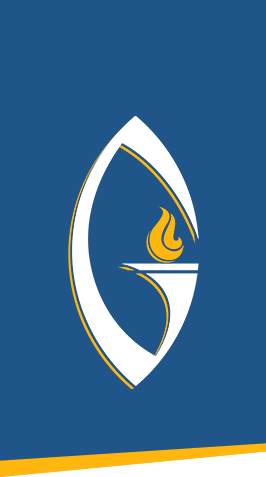## MATH 213: Math Concepts and Applications

MATH 213: Math Concepts and Applications Covers a variety of topics in math which may vary but generally are chosen from areas such as problem solving, algebra review, elementary statistics and probability, consumer math, voting methods, finance, number sense,...

## MATH 122: Statistics

MATH 122: Statistics Measures of central tendency and variability, probability, interval estimation, hypothesis testing, correlation and regression, and introduction to nonparametric techniques. 3 credits

## MATH 101: College Algebra

MATH 101: College Algebra Basic concepts of algebra, including real numbers, linear equations, polynomials, factoring, rational algebraic expressions, irrational equations, exponents and radicals, logarithmic and exponential functions, graphs of equations, quadratic...

## COMP 119: Technology Applications for Business

COMP 119: Technology Applications for Business This course introduces students to a range of technologies and tools for business use. Coverage includes the basic MS Office suite (Access, Excel, Word), as well as new and emerging technologies such as cloud and mobile...

## COMP 111: Introduction to Computer Technology

COMP 111: Introduction to Computer Technology This course introduces students to basic computer concepts, computer applications, and various productivity software applications (word processing, spreadsheet, database and presentation) as well as basic website design....